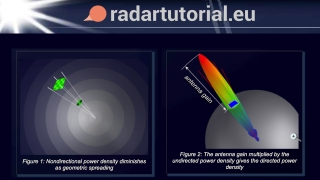# The Radar Range Equation - radartutorial.euBeginner
Article / Blog
Theory

This article describes the radar range equation. The radar range equation represents the physical dependences of the transmit power, which is the wave propagation up to the receiving of the echo signals. The power Pe returning to the receiving antenna is given by the radar equation, depending on the transmitted power PS, the slant range R, and the reflecting characteristics of the aim (described as the radar cross-section σ). At the known sensibility of the radar receiver, the radar equation determines the achieved by a given radar theoretically maximum range. Furthermore one can assess the performance of the radar set with the radar range equation (or shorter: the radar equation).

This resource is included in the following topics and journeys: